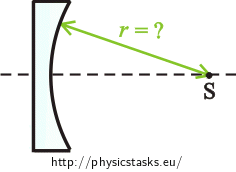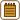## A Plano-concave Lens

A plano-concave lens made of glass of refractive index of $$1{.}5$$ is located in the air. Its focal length is $$−10 \mathrm{cm}$$. Determine its radius of curvature.• #### Notation

 $$n_{g}=1{.}5$$ refractive index of glass $$f=10 \mathrm{cm}$$ focal length $$r= ?$$ radius of curvature
• #### Hint 1

Each lens has two radii of curvature. Why are we looking only for the value of one of them in this case?

• #### Solution of Hint 1

We already know the value of the radius of curvature $$r_{1}$$ of the plane (first) surface because it is approaching infinity. That is why we are only looking for the radius of curvature of the concave (second) surface $$r_{2}=r$$.

• #### Hint 2

Let us assume that it is a thin lens. The radii of curvature affect the focal length of the lens. Could you describe this fact mathematically?

• #### Hint 3

How should we adjust the lensmaker’s equation, so that the focal length depends on only the one radius of curvature $$r_{2}=r$$? Then express this radius and calculate it.

• #### Overall Solution

The lensmaker’s equation for a thin lens

Change in the focal length of the lens $$f$$ in dependence on the changes in the radii of curvature of optical surface $$r_{1}$$ and $$r_{2}$$ is defined by the lensmaker’s equation. Let’s assume that we have a thin lens: $\frac{1}{f}=\left(\frac{n_{g}}{n_{a}}−1\right)\left(\frac{1}{r_{1}}+\frac{1}{r_{2}}\right),$ where $$n_{g}$$ is the refractive index of glass and $$n_{a}$$ is the refractive index of air.

Radius of curvature $$r_{1}$$ is approaching infinity, which means that fraction $$\frac{1}{r_{1}}$$ is approaching zero. Therefore, this fraction will „disappear“ from the equation:

$\frac{1}{f}=\left(\frac{n_{g}}{n_{a}}−1\right)\frac{1}{r_{2}}.$

Denote radius of curvature $$r_{2}$$ by $$r$$ ($$r_{2}=r$$):

$\frac{1}{f}=\left(\frac{n_{g}}{n_{a}}−1\right)\frac{1}{r}.$

Multiply both sides of the equation by $$r\cdot f$$: $r=\left(\frac{n_{g}}{n_{a}}−1\right)f.$

Numerical solution

We know from the task assignment:

$$n_{g}=1{.}5$$ (refractive index of glass)

$$f=−10 \mathrm{cm}$$ (focal length of the lens)

We look up the value of the refractive index of air in the Tables:

$$n_{a}=1{.}0$$

We substitute for the values:

$$r=\left(\frac{n_{g}}{n_{a}}−1\right)f=\left(\frac{1{.}5}{1{.}0}−1\right)\cdot \left(−10\right) \mathrm{cm}=0{.}5\cdot \left(−10\right) \mathrm{cm}=−5 \mathrm{cm}.$$

It is the radius of curvature of the concave surface, therefore, its value is negative.

The value of the radius of curvature of the plano-concave lens is $$−5 \mathrm{cm}$$.